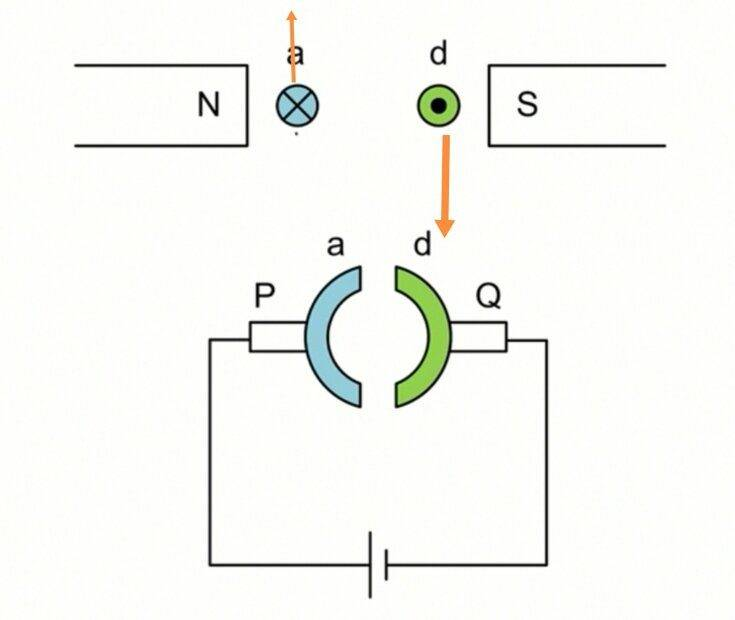# Electromagnetism and the movement of particles

• ellieee
On your diagram, the field direction is left to right. The direction is not into/out of the paper. Using dots and crosses to show the field direction would be completely wrong in this situation.Remember, your diagram is a simplified cross-section of this sort of setup:https://docbrown.info/ephysics/ephyspics/electromag07.gif

#### ellieee

Homework Statement
are the orange arrows indicating the correct direction that each particle moves in?
Relevant Equations
-ellieee said:
Homework Statement:: are the orange arrows indicating the correct direction that each particle moves in?
Relevant Equations:: -

View attachment 283900
PF rules require that you need to make some effort yourself before we can help. Do you think the orange arrows are correct? What's your reasoning?

Also, I think there are no 'particles' in the top diagram, 'a' and 'd' are meant to be cross-sections through wires carrying current. Do you know why 'a' has a cross and 'd' has a dot?

•Delta2
Steve4Physics said:
Do you think the orange arrows are correct? What's your reasoning?
yep I think they are. my reasoning is: a cross represents the magnetic field traveling into the plane of the paper, thus the index finger is pointing into the paper. then, the current is flowing from right to left(because the positive terminal of the battery is on the right while negative terminal is on the left) , so the middle finger is pointing to the right. thus, the thumb is pointing forward, which means the force is traveling upwards, vice versa for the dot.

ellieee said:
yep I think they are. my reasoning is: a cross represents the magnetic field traveling into the plane of the paper, thus the index finger is pointing into the paper. then, the current is flowing from right to left(because the positive terminal of the battery is on the right while negative terminal is on the left) , so the middle finger is pointing to the right. thus, the thumb is pointing forward, which means the force is traveling upwards, vice versa for the dot.
There are some mistakes.

The lower part of the diagram (commutator) in Post #1 is not needed. So I am referring only to the upper part of the diagram.

1. In the space between the magnetic poles, the direction of the magnetic field is left to right (from N-pole to S-pole). The ‘X’ is not being used to show you the field-direction in this question – see note 2.

2. ‘a’ and ‘d’ are cross-sections through wire (the wire is probably meant to be a loop in a simple motor).

The cross in circle 'a' represents the (conventional) current flowing through wire ‘a’ into the paper (away from you).

The dot in circle 'd' represents the (conventional) current flowing through wire ‘d’ out of the paper (towards you).

3. You can now apply Fleming’s left hand rule.

Steve4Physics said:
The ‘X’ is not being used to show you the field-direction in this question – see note 2.
how do you know when the cross/dot is used or not used to show field-direction?
I saw note 2 already but how does it prove that the cross is not used to show field-direction?

ellieee said:
how do you know when the cross/dot is used or not used to show field-direction?
I saw note 2 already but how does it prove that the cross is not used to show field-direction?
When North and South poles are shown, you know the field lines go North to South. So the field lines in the gap between the poles are lines drawn like this: https://physicsigcse.files.wordpress.com/2016/04/uniformfields.jpg and you know the direction is left to right. (In real life, the field lines are curved,so this is a simplification).

That means we can use a circle with a dot or cross to represent a cross-section of a current going in or out.

As a general rule, dots and crosses for fields are only used when the N and S poles are above/below the paper.

So you have to think about each diagram to fully understand what is being shown.

Steve4Physics said:
As a general rule, dots and crosses for fields are only used when the N and S poles are above/below the paper
so in my diagram, the north and south poles are lying on the same plane as the dots and crosses that's why the cross/dot are not used to show field-direction?

ellieee said:
so in my diagram, the north and south poles are lying on the same plane as the dots and crosses that's why the cross/dot are not used to show field-direction?
In your diagram the north and south poles are in the page(paper) plane if that's what you mean.

ellieee said:
so in my diagram, the north and south poles are lying on the same plane as the dots and crosses that's why the cross/dot are not used to show field-direction?
On your diagram, the field direction is left to right. The direction is not into/out of the paper. Using dots and crosses to show the field direction would be completely wrong in this situation.

Remember, your diagram is a simplified cross-section of this sort of setup: https://docbrown.info/ephysics/ephyspics/electromag07.gif

You need to think in 3 dimensions so you can relate your diagram to the one shown in the above link.